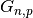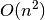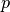Warning

This documents an unmaintained version of NetworkX. Please upgrade to a maintained version and see the current NetworkX documentation.

# binomial_graph¶

binomial_graph(n, p, seed=None, directed=False)

Returns arandom graph, also known as an Erdős-Rényi graph or a binomial graph.

Themodel chooses each of the possible edges with probability p.

The functions binomial_graph() and erdos_renyi_graph() are aliases of this function.

Parameters: n (int) – The number of nodes. p (float) – Probability for edge creation. seed (int, optional) – Seed for random number generator (default=None). directed (bool, optional (default=False)) – If True, this function returns a directed graph.

Notes

This algorithm runs intime. For sparse graphs (that is, for small values of), fast_gnp_random_graph() is a faster algorithm.

References

  Erdős and A. Rényi, On Random Graphs, Publ. Math. 6, 290 (1959).
  Gilbert, Random Graphs, Ann. Math. Stat., 30, 1141 (1959).